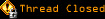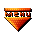# Topic awaiting preservation: I cannot get alert...Boolean Problem2 (Page 1 of 1)Hiroki
Paranoid (IV) Inmate

From: NZ
Insane since: Dec 2002posted 08-07-2003 02:03

Hi, guys. Could I ask you other question?
Here is my exercise:

quote:
Write a progarm to calculate the interest on a bank account. The interest is:
0% on balances <\$1000
1% on balances <=\$1000 and <\$5000
1.5% on balances <=\$5000.
As user error detection, if user enter negative number, get alert saying "Work hard!".

I cannot understand why this code does not work. Then I modified like secode code. Works fine. But I think logically first one is good way to deal with this question. Do you see anything wrong???

code:
```function cal(){

if(5000<=form1.acc.value){

form1.int.value=Math.round(0.015*(form1.acc.value-5000)+40);

}else if((1000<=form1.acc.value) && (form1.acc.value<5000)){

form1.int.value=Math.round(0.01*(form1.acc.value-1000));

}else if((0<=form1.int.value)&&(form1.int.value<1000)){

form1.int.value=0;

}else{

}```

code:
```function cal(){

if(form1.acc.value<0){

}

if(5000<=form1.acc.value){

form1.int.value=Math.round(0.015*(form1.acc.value-5000)+40);

}else if((1000<=form1.acc.value) && (form1.acc.value<5000)){

form1.int.value=Math.round(0.01*(form1.acc.value-1000));

}else{

form1.int.value=0;

}```

Thanks.

Hiroki Kozai

Slime
Lunatic (VI) Mad Scientist

From: Massachusetts, USA
Insane since: Mar 2000posted 08-07-2003 04:36

if(5000<=form1.acc.value)

Consider if the input's value is, say, 1000:

if (5000 <= 1000)

5000 is not less than or equal to 1000, so the if block won't be entered.

Are you sure you didn't mean

if (form1.acc.value <= 5000)

?

Hiroki
Paranoid (IV) Inmate

From: NZ
Insane since: Dec 2002posted 08-08-2003 00:08

Yeah, I am sure about that, Slime.
How are you today?
Well, I fixed this problem.
You told me to put parseInt when I deal with If statement.
I did that.
Then worked.
Many thanks.
Cheers.

Hiroki Kozai